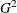### Log-Linear Model Analysis

When the response functions are the default generalized logits, then inclusion of the keyword _RESPONSE_ in every effect in the right side of the MODEL statement fits a log-linear model. The keyword _RESPONSE_ tells PROC CATMOD that you want to model the variation among the dependent variables. You then specify the actual model in the LOGLIN statement.

When you perform log-linear model analysis, you can request weighted least squares estimates, maximum likelihood estimates, or both. By default, PROC CATMOD calculates maximum likelihood estimates when the default response functions are used. The following table provides appropriate MODEL statements for the combinations of types of estimates:

Estimation Desired

MODEL Statement

Maximum likelihood(Newton-Raphson)

`model a*b=_response_;`

Maximum likelihood(Iterative Proportional Fitting)

`model a*b=_response_ / ml=ipf;`

Weighted least squares

`model a*b=_response_ / wls;`

Maximum likelihood and weighted least squares

`model a*b=_response_ / wls ml;`

Caution: Sampling zeros in the input data set should be specified with the ZERO= option to ensure that these sampling zeros are not treated as structural zeros. Alternatively, you can replace cell counts for sampling zeros with some positive number close to zero (such as 1E–20) in a DATA step. Data containing sampling zeros should be analyzed with maximum likelihood estimation. See the section Cautions and Example 30.5 for further information and an illustration that uses both cell count data and raw data.

#### One Population

The usual log-linear model analysis has one population, which means that all of the variables are dependent variables. For example, the following statements yield a maximum likelihood analysis of a saturated log-linear model for the dependent variables `r1` and `r2`:

```proc catmod;
weight wt;
model r1*r2=_response_;
loglin r1|r2;
run;
```

If you want to fit a reduced model with respect to the dependent variables (for example, a model of independence or conditional independence), specify the reduced model in the LOGLIN statement. For example, the following statements yield a main-effects log-linear model analysis of the factors `r1` and `r2`:

```proc catmod;
weight wt;
model r1*r2=_response_ / pred;
loglin r1 r2;
run;
```

The output includes Wald statistics for the individual effects `r1` and `r2`, as well as predicted cell probabilities. Moreover, the goodness-of-fit statistic is the likelihood ratio test for the hypothesis of independence between `r1` and `r2` or, equivalently, a test of `r1`*`r2`.

#### Multiple Populations

You can do log-linear model analysis with multiple populations by using a POPULATION statement or by including effects on the right side of the MODEL statement that contain independent variables. Each effect must include the _RESPONSE_ keyword.

For example, suppose the dependent variables `r1` and `r2` are dichotomous, and the independent variable `group` has three levels. Then the following statements specify a saturated model (three degrees of freedom for _RESPONSE_ and six degrees of freedom for the interaction between _RESPONSE_ and `group`):

```proc catmod;
weight wt;
model r1*r2=_response_ group*_response_;
loglin r1|r2;
run;
```

From another point of view, _RESPONSE_*`group` can be regarded as a main effect for `group` with respect to the three response functions, while _RESPONSE_ can be regarded as an intercept effect with respect to the functions. In other words, the following statements give essentially the same results as the logistic analysis:

```proc catmod;
weight wt;
model r1*r2=group;
run;
```

The ability to model the interaction between the independent and the dependent variables becomes particularly useful when a reduced model is specified for the dependent variables. For example, the following statements specify a model with two degrees of freedom for _RESPONSE_ (one for `r1` and one for `r2`) and four degrees of freedom for the interaction of _RESPONSE_*`group`:

```proc catmod;
weight wt;
model r1*r2=_response_ group*_response_;
loglin r1 r2;
run;
```

The likelihood ratio goodness-of-fit statistic (three degrees of freedom) tests the hypothesis that `r1` and `r2` are independent in each of the three groups.

#### Iterative Proportional Fitting

You can use the iterative proportional fitting (IPF) algorithm to fit a hierarchical log-linear model with no independent variables and no population variables.

The advantage of IPF over the Newton-Raphson (NR) algorithm and over the weighted least squares (WLS) method is that, when the contingency table has several dimensions and the parameter vector is large, you can obtain the log likelihood, the goodness-of-fit, and the predicted frequencies or probabilities without performing potentially expensive parameter estimation and covariance matrix calculations. This enables you to do the following:

• compare two models by computing the likelihood ratio statistics to test the significance of the contribution of the variables in one model that are not in the other model

• compute predicted values of the cell probabilities or frequencies for the final model

Each iteration of the IPF algorithm is generally faster than an iteration of the NR algorithm; however, the IPF algorithm converges to the MLEs more slowly than the NR algorithm. Both NR and WLS are more general methods that are able to perform more complex analyses than IPF can.